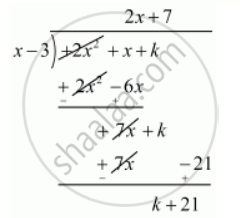Advertisement Remove all ads

# For What Value of K, is 3 a Zero of the Polynomial 2x2 + X + K? - Mathematics

Sum

For what value of k, is 3 a zero of the polynomial 2x2 + x + k?

Advertisement Remove all ads

#### Solution

We know that if  x = a is zero polynomial, and then  x - a is a factor of  f(x)

Since 3 is zero of   f (x)

Therefore x - 3 is a factor of  f(x)

Now, we divide f(x)=2x^2+ x+ k  by g (x) = x-3  to find the value of kNow, remainder = 0

k + 21 = 0

 k = -21

Hence, the value of k is = -21

Is there an error in this question or solution?
Advertisement Remove all ads

#### APPEARS IN

RD Sharma Class 10 Maths
Chapter 2 Polynomials
Q 33 | Page 60
Advertisement Remove all ads

#### Video TutorialsVIEW ALL 

Advertisement Remove all ads
Share
Notifications

View all notifications

Forgot password?